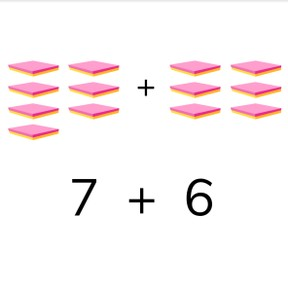8,000 schools use Gynzy92,000 teachers use Gynzy1,600,000 students use Gynzy

## General

Students learn to add to 20.

1.OA.C

## Relevance

It is important to be able to add to 20, so you know how many you have together.

## Introduction

The interactive whiteboard shows a number sequence to twenty, with four numbers missing. Students must determine what the missing numbers are from the number bank on the right and drag them to their spots in the sequence.

## Development

Check that students understand adding to 20 by asking the following questions:
- What is useful if you want to add on the number line?
- What do you look at if you want to solve a story problem?
- How do you calculate the sum of 8+7?

## Quiz

Students first practice adding two addends with visual support. Next they are only given the addition problem and must calculate the total. They are then given a story problem. Ask students what the addition problem is and how they solve it.

## Closing

Repeat the lesson goal and why it is important. The interactive whiteboard shows a number of baby chicks as well as a nest with baby chicks just hatching. The students must come up with the addition problem that represents the image, and then calculate the total. You can ask students how they determined the problem as well as how they calculated the total. They do the same with the image of glasses of juice.

## Teaching tips

Students who have difficulty with this can be supported by the use of blocks or other manipulatives.

## Instruction materials

Optional: blocks or manipulatives

### The online teaching platform for interactive whiteboards and displays in schools

• Save time building lessons

• Manage the classroom more efficiently

• Increase student engagement